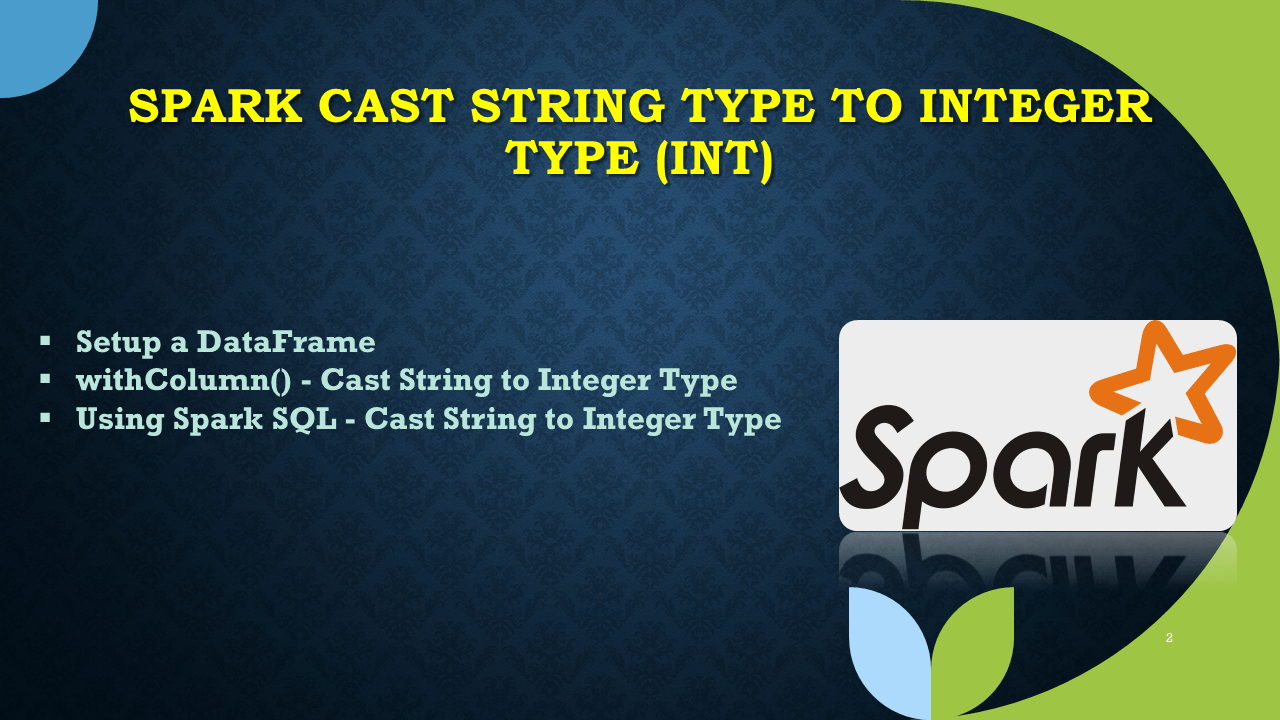# Spark Cast String Type to Integer Type (int)

In Spark SQL, in order to convert/cast String Type to Integer Type (int), you can use `cast()` function of Column class, use this function with withColumn(), select(), selectExpr() and SQL expression. This function takes the argument string representing the type you wanted to convert or any type that is a subclass of DataType.

Key points

• `cast()`cast() is a function from `Column` class that is used to convert the column into the other datatype.
• When Spark unable to convert into a specific type, cast() function returns a null value.
• This function takes the argument string representing the type you wanted to convert or any type that is a subclass of DataType.
• Spark SQL takes the different syntax `INETGER(String column)` to cast types.

Following are some Spark examples that change/convert String Type to Integer Type (int).

``````
import org.apache.spark.sql.functions.col
import org.apache.spark.sql.types.IntegerType

// Convert String to Integer Type
df.withColumn("salary",col("salary").cast(IntegerType))
df.withColumn("salary",col("salary").cast("int"))
df.withColumn("salary",col("salary").cast("integer"))

// Using select
df.select(col("salary").cast("int").as("salary"))

//Using selectExpr()

//Using with spark.sql()
spark.sql("SELECT cast(salary as int) salary, BOOLEAN(isGraduated),gender from CastExample")
``````

## 1. Setup a DataFrame

Let’s run with some examples.

``````
val spark = SparkSession.builder
.master("local")
.appName("SparkByExamples.com")
.getOrCreate()

val simpleData = Seq(("James",34,"true","M","3000.6089"),
("Michael",33,"true","F","3300.8067"),
("Robert",37,"false","M","5000.5034")
)

import spark.implicits._
df.printSchema()
``````

Outputs below schema. Note that column `salary` is a string type.

## 2. withColumn() – Cast String to Integer Type

First will use Spark DataFrame withColumn() to cast the `salary` column from String Type to Integer Type, this withColumn() transformation takes the column name you wanted to convert as a first argument and for the second argument you need to apply the casting method `cast()`.

``````
import org.apache.spark.sql.functions.col
import org.apache.spark.sql.types.IntegerType

// Convert String to Integer Type
val df2= df.withColumn("salary",col("salary").cast(IntegerType))
df2.printSchema()
df2.show()
``````

Outputs below schema & DataFrame.

Alternatively, you can also change the data type using below.

``````
df.withColumn("salary",col("salary").cast("int"))
df.withColumn("salary",col("salary").cast("integer"))
``````

## 3. Using select() Example

Following example uses selectExpr() transformation of SataFrame on order to change the data type.

``````
// Using select
df.select(col("salary").cast("int").as("salary")).printSchema()

//Using selectExpr()
df.selectExpr("cast(salary as int) salary").printSchema()
``````

## 4. Using Spark SQL – Cast String to Integer Type

Spark SQL expression provides data type functions for casting and we can’t use `cast()` function. Below `INT(string column name)` is used to convert to Integer Type.

``````
df.createOrReplaceTempView("CastExample")
df4=spark.sql("SELECT firstname,age,isGraduated,INT(salary) as salary from CastExample")
``````

## 5. Conclusion

In this simple Spark article, I have covered how to convert the DataFrame column from String Type to Integer Type using cast() function and applying it with withColumn(), select(), selectExpr() and finally Spark SQL table.

Happy Learning !!

### Naveen (NNK)

Naveen (NNK) is a Data Engineer with 20+ years of experience in transforming data into actionable insights. Over the years, He has honed his expertise in designing, implementing, and maintaining data pipelines with frameworks like Apache Spark, PySpark, Pandas, R, Hive and Machine Learning. Naveen journey in the field of data engineering has been a continuous learning, innovation, and a strong commitment to data integrity. In this blog, he shares his experiences with the data as he come across. Follow Naveen @ @ LinkedIn

### This Post Has 6 Comments

1.Anonymous

thank you

2.Anonymous

thank you

3.Sriram

Does this “org.apache.spark.sql.types.IntegerType” is not supported in the recent versions of Spark?

4.Sriram

Does this “org.apache.spark.sql.types.IntegerType” is not supported in the recent versions of Spark?